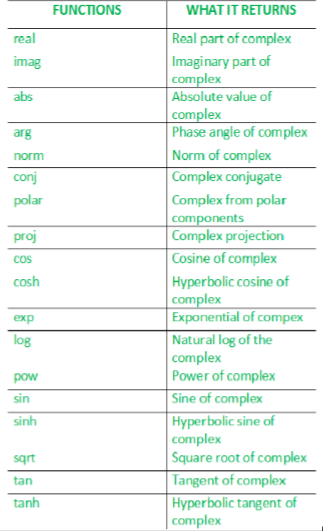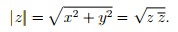# Complex numbers in C++ | Set 1

• Difficulty Level : Medium
• Last Updated : 06 Jul, 2017

The complex library implements the complex class to contain complex numbers in cartesian form and several functions and overloads to operate with them.Want to learn from the best curated videos and practice problems, check out the C++ Foundation Course for Basic to Advanced C++ and C++ STL Course for foundation plus STL.  To complete your preparation from learning a language to DS Algo and many more,  please refer Complete Interview Preparation Course.
• real() – It returns the real part of the complex number.
• imag() – It returns the imaginary part of the complex number.
 `// Program illustrating the use of real() and ``// imag() function``#include      `` ` `// for std::complex, std::real, std::imag``#include       ``using` `namespace` `std;`` ` `// driver function``int` `main()``{    ``  ``// defines the complex number: (10 + 2i)``  ``std::complex<``double``> mycomplex(10.0, 2.0);`` ` `  ``// prints the real part using the real function``  ``cout << ``"Real part: "` `<< real(mycomplex) << endl;``  ``cout << ``"Imaginary part: "` `<< imag(mycomplex) << endl;``  ``return` `0;``}`

Output:

```Real part: 10
Imaginary part: 2
```
• abs() – It returns the absolute of the complex number.
• arg() – It returns the argument of the complex number.
 `// Program illustrating the use of arg() and abs()``#include      `` ` `// for std::complex, std::abs, std::atg``#include  ``using` `namespace` `std;`` ` `// driver function``int` `main ()``{    ``  ``// defines the complex number: (3.0+4.0i)``  ``std::complex<``double``> mycomplex (3.0, 4.0);`` ` `  ``// prints the absolute value of the complex number``  ``cout << ``"The absolute value of "` `<< mycomplex << ``" is: "``;``  ``cout << ``abs``(mycomplex) << endl;``   ` `  ``// prints the argument of the complex number``  ``cout << ``"The argument of "` `<< mycomplex << ``" is: "``;``  ``cout << arg(mycomplex) << endl;`` ` `  ``return` `0;``}`

Output:

```The absolute value of (3,4) is: 5
The argument of (3,4) is: 0.927295
```
• polar() – It constructs a complex number from magnitude and phase angle.

real = magnitude*cosine(phase angle)
imaginary = magnitude*sine(phase angle)

 `// Program illustrating the use of polar()``#include      `` ` `// std::complex, std::polar``#include ``using` `namespace` `std;`` ` `// driver function``int` `main ()``{``  ``cout << ``"The complex whose magnitude is "` `<< 2.0;``  ``cout << ``" and phase angle is "` `<< 0.5;``   ` `  ``// use of polar()``  ``cout << ``" is "` `<< polar (2.0, 0.5) << endl;`` ` `  ``return` `0;``}`

Output:

```The complex whose magnitude is 2 and phase angle is 0.5 is (1.75517,0.958851)
```
• norm() – It is used to find the norm(absolute value) of the complex number. If z = x + iy is a complex number with real part x and imaginary part y, the complex conjugate of z is defined as z'(z bar) = x – iy, and the absolute value, also called the norm, of z is defined as :`// example to illustrate the use of norm()``#include      `` ` `// for std::complex, std::norm``#include  ``using` `namespace` `std;`` ` `// driver function``int` `main ()``{    ``  ``// initializing the complex: (3.0+4.0i)``  ``std::complex<``double``> mycomplex (3.0, 4.0);`` ` `  ``// use of norm()``  ``cout << ``"The norm of "` `<< mycomplex << ``" is "` `       ``<< norm(mycomplex) <

Output:

```The norm of (3,4) is 25.
```
• conj() – It returns the conjugate of the complex number x. The conjugate of a complex number (real,imag) is (real,-imag).
 `// Illustrating the use of conj()``#include  ``using` `namespace` `std;`` ` `// std::complex, std::conj``#include       `` ` `// driver program``int` `main ()``{``  ``std::complex<``double``> mycomplex (10.0,2.0);`` ` `  ``cout << ``"The conjugate of "` `<< mycomplex << ``" is: "``;``   ` `  ``// use of conj()``  ``cout << conj(mycomplex) << endl;``  ``return` `0;``}`

Output:

``` The conjugate of (10,2) is (10,-2)
```
• proj() – It returns the projection of z(complex number) onto the Riemann sphere. The projection of z is z, except for complex infinities, which are mapped to the complex value with a real component of INFINITY and an imaginary component of 0.0 or -0.0 (where supported), depending on the sign of the imaginary component of z.
 `// Illustrating the use of proj()`` ` `#include ``using` `namespace` `std;`` ` `// For std::complex, std::proj``#include ``  ` `// driver program``int` `main()``{``    ``std::complex<``double``> c1(1, 2);``    ``cout << ``"proj"` `<< c1 << ``" = "` `<< proj(c1) << endl;``  ` `    ``std::complex<``double``> c2(INFINITY, -1);``    ``cout << ``"proj"` `<< c2 << ``" = "` `<< proj(c2) << endl;``  ` `    ``std::complex<``double``> c3(0, -INFINITY);``    ``cout << ``"proj"` `<< c3 << ``" = "` `<< proj(c3) << endl;``}`

Output:

```proj(1,2) = (1,2)
proj(inf,-1) = (inf,-0)
proj(0,-inf) = (inf,-0)
```
• sqrt() – Returns the square root of x using the principal branch, whose cuts are along the negative real axis.
 `// Illustrating the use of sqrt()``#include ``using` `namespace` `std;`` ` `// For std::ccomplex, stdc::sqrt``#include ``  ` `// driver program``int` `main()``{    ``    ``// use of sqrt()``    ``cout << ``"Square root of -4 is "``         ``<< ``sqrt``(std::complex<``double``>(-4, 0)) << endl``         ``<< ``"Square root of (-4,-0), the other side of the cut, is "``         ``<< ``sqrt``(std::complex<``double``>(-4, -0.0)) << endl;``}`

Output:

```Square root of -4 is (0,2)
Square root of (-4,-0), the other side of the cut, is (0,-2)
```

Next article: Complex numbers in C++ | Set 2

This article is contributed by Shambhavi Singh. If you like GeeksforGeeks and would like to contribute, you can also write an article using contribute.geeksforgeeks.org or mail your article to contribute@geeksforgeeks.org. See your article appearing on the GeeksforGeeks main page and help other Geeks.